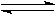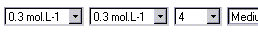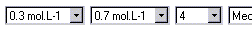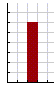Chemistry Le Chatelier's principle: effect of concentration

This model (below) demonstrates the attainment of equilibrium in a model chemical reaction.The way in which the position of equilibrium is affected by altering the conditions is summarised by Le Chatelier's principle.

 If the conditions of a system at equilibrium are changed, the system moves in such a way as to oppose the effects of that change.

Thus increasing the concentration of reactants in a reaction results in the formation of more product molecules so that the value of Kc, the equilibrium constant, is maintained.

For the reaction:

A + BC + D

the equilibrium constant, Kc is given by:

Kc = [C] x [D] / [A] x [B]

where [A] is the concentration of reactant A at equilibrium etc.

The model below can be used to demonstrate the effect of changing reactant concentration. Increasing one reactant concentration will result in the formation of more product so that the value of the equilibrium constant is maintained.

 The initial conditions for the model are an inital concentration for reactant A and reactant B of 0.3 mol.L-1, a molecule size of 4 and a speed of slow. The initial conditions can be changed using the drop down menus.

A suitable place to start is by comparing the following two models runs:

Run 1:Run 2:You will find that when equilibrium is attained (after about 60 seconds) a greater concentration of product is formed when the concentration of reactant B is raised from 0.3 mol-L-1 to 0.7 mol.L-1.

Notes on using the applet:

 the model is set to stop after a maximum running time of 5 minutes the particles in the model have a range of velocities only those collisions with a kinetic energy greater than a minimum activation energy, Ea, lead to a reaction. Collisions with a kinetic energy below this minimum are not successful the Ea value and the rate constant for the forward reaction is set to be the same as the reverse reaction. This leads to an equlibrium constant for the model of Kc = 1.00. the model represents concentrations in the range 0.1 to 0.9 mol.L-1 with a number of "molecules" in the animation. This representation is one of proportion as clearly it is not possible to display of the order 1e22 to 9e22 molecules on a PC. the PC calculates data based on the number of displayed molecules.Only super-computers can approach the computing power necessary to model between 1e22 to 9e22 molecules. This means that at equilibrium there is rather more observable fluctuation in the number of each type of molecule than would be observed in a real chemical process. To overcome this fluctuation, the model calculates a time averaged value for the concentration display. it is possible to use the equilibrium concentration data generated by the model to calculate an equilibrium constant for the model. Students and teachers may find some lack of precision in the measurements due to the constraints discussed above relating to PC processing power. These errors may be reduced in the normal way by making replicate measurments from the model.